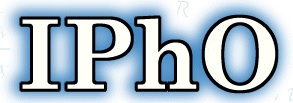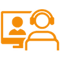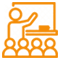Alert: You are not allowed to copy content or view sourceThe International Physics Olympiad (IPhO) is an international physics competition for secondary school pupils. The first such competition was organised by Prof. Czesław Ścisłowski in Warsaw (Poland) in 1967. Since that era the International Physics Olympiads have been organised, with few variations that will be discussed later, in a different country every year.

## SYLLABUS:

1. Theoretical Part

The first column contains the main entries while the second column contains comments and remarks if necessary.

1. Mechanics
 a) Foundation of kinematics of a point mass Vector description of the position of the point mass, velocity and acceleration as vectors b) Newton's laws, inertial systems Problems may be set on changing mass c) Closed and open systems, momentum and energy, work, power d) Conservation of energy, conservation of linear momentum, impulse e) Elastic forces, frictional forces, the law of gravitation, potential energy and work in a gravitational field Hooke's law, coefficient of friction (F/R = const), frictional forces, static and kinetic, choice of zero of potential energy f) Centripetal acceleration, Kepler's laws
1. Mechanics of Rigid Bodies
 a) Statics, center of mass, torque Couples, conditions of equilibrium of bodies b) Motion of rigid bodies, translation, rotation, angular velocity, angular acceleration, conservation of angular momentum Conservation of angular momentum about fixed axis only c) External and internal forces, equation of motion of a rigid body around the fixed axis, moment of inertia, kinetic energy of a rotating body Parallel axes theorem (Steiner's theorem), additivity of the moment of inertia d) Accelerated reference systems, inertial forces Knowledge of the Coriolis force formula is not required
1. Hydromechanics

No specific questions will be set on this but students would be expected to know the elementary concepts of pressure, buoyancy and the continuity law.

1. Thermodynamics and Molecular Physics
• Oscillations and waves
• Electric Charge and Electric Field
• Current and Magnetic Field
• Electromagnetic waves
• Quantum Physics
• Relativity
• Matter

B. Practical PartThe Theoretical Part of the Syllabus provides the basis for all the experimental problems. The experimental problems given in the experimental contest should contain measurements

 a) Internal energy, work and heat, first and second laws of thermodynamics Thermal equilibrium, quantities depending on state and quantities depending on process b) Model of a perfect gas, pressure and molecular kinetic energy, Avogadro's number, equation of state of a perfect gas, absolute temperature Also molecular approach to such simple phenomena in liquids and solids as boiling, melting etc. c) Work done by an expanding gas limited to isothermal and adiabatic processes Proof of the equation of the adiabatic process is not required d) The Carnot cycle, thermodynamic efficiency, reversible and irreversible processes, entropy (statistical approach), Boltzmann factor Entropy as a path independent function, entropy changes and reversibility, quasistatic processes a) Harmonic oscillations, equation of harmonic oscillation Solution of the equation for harmonic motion, attenuation and resonance -qualitatively b) Harmonic waves, propagation of waves, transverse and longitudinal waves, linear polarization, the classical Doppler effect, sound waves Displacement in a progressive wave and understanding of graphical representation of the wave, measurements of velocity of sound and light, Doppler effect in one dimension only, propagation of waves in homogeneous and isotropic media, reflection and refraction, Fermat's principle c) Superposition of harmonic waves, coherent waves, interference, beats, standing waves Realization that intensity of wave is proportional to the square of its amplitude. Fourier analysis is not required but candidates should have some understanding that complex waves can be made from addition of simple sinusoidal waves of different frequencies. Interference due to thin films and other simple systems (final formulae are not required), superposition of waves from secondary sources (diffraction) a) Conservation of charge, Coulomb's law b) Electric field, potential, Gauss' law Gauss' law confined to simple symmetric systems like sphere, cylinder, plate etc., electric dipole moment c) Capacitors, capacitance, dielectric constant, energy density of electric field a) Current, resistance, internal resistance of source, Ohm's law, Kirchhoff's laws, work and power of direct and alternating currents, Joule's law Simple cases of circuits containing non-ohmic devices with known V-I characteristics b) Magnetic field (B) of a current, current in a magnetic field, Lorentz force Particles in a magnetic field, simple applications like cyclotron, magnetic dipole moment c) Ampere's law Magnetic field of simple symmetric systems like straight wire, circular loop and long solenoid d) Law of electromagnetic induction, magnetic flux, Lenz's law, self-induction, inductance, permeability, energy density of magnetic field e) Alternating current, resistors, inductors and capacitors in AC-circuits, voltage and current (parallel and series) resonances Simple AC-circuits, time constants, final formulae for parameters of concrete resonance circuits are not required a) Oscillatory circuit, frequency of oscillations, generation by feedback and resonance b) Wave optics, diffraction from one and two slits, diffraction grating,resolving power of a grating, Bragg reflection, c) Dispersion and diffraction spectra, line spectra of gases d) Electromagnetic waves as transverse waves, polarization by reflection, polarizers Superposition of polarized waves e) Resolving power of imaging systems f) Black body, Stefan-Boltzmanns law Planck's formula is not required a) Photoelectric effect, energy and impulse of the photon Einstein's formula is required b) De Broglie wavelength, Heisenberg's uncertainty principle a) Principle of relativity, addition of velocities, relativistic Doppler effect b) Relativistic equation of motion, momentum, energy, relation between energy and mass, conservation of energy and momentum a) Simple applications of the Bragg equation b) Energy levels of atoms and molecules (qualitatively), emission, absorption, spectrum of hydrogen like atoms c) Energy levels of nuclei (qualitatively), alpha-, beta- and gamma-decays, absorption of radiation, halflife and exponential decay, components of nuclei, mass defect, nuclear reactions

## Explore

#### SchoolPlus Program

Yearlong program for Olympiads preparation & to build necessary skills for future.

Explore MoreExplore MoreExplore MoreAssess your performance by taking topic-wise and full length mock tests.

Explore MoreExplore MoreExplore More